• 数字图像处理实验——Python语言实现 实验一：数字图像处理入门 实验二：直方图均衡 实验三：线性平滑和锐化——掩模法 实验四：非线性平滑——中值滤波 实验五：非线性锐化——梯度法 GitHub地址：...
数字图像处理实验——Python语言实现
实验一：数字图像处理入门
实验二：直方图均衡
实验三：线性平滑和锐化——掩模法
实验四：非线性平滑——中值滤波
实验五：非线性锐化——梯度法
GitHub地址：https://github.com/liuminxuan2016/DIPexperiment


展开全文• 数字图像处理实验之直方图均衡化算法完成测试图像的增强python实现 一、实验要求 在熟悉直方图均衡化的数学原理以及数字图像下直方图均衡化步骤基础上，能够利用python语言编写图像直方图均衡化算法。注意，不能简单...
数字图像处理实验之直方图均衡化算法完成测试图像的增强python实现
一、实验要求
在熟悉直方图均衡化的数学原理以及数字图像下直方图均衡化步骤基础上，能够利用python语言编写图像直方图均衡化算法。注意，不能简单使用exposure模块中的equalize_hist()函数来完成直方图均衡化，需自己编写直方图均衡化算法完成测试图像的增强。按实验内容及步骤完成操作，完成实验报告，所有实验环节均由每位学生独立完成，严禁抄袭他人实验结果。
二、测试图像三、实验步骤
1.理解并初步建立直方图均衡化算法
直方图均衡化是使图像直方图变得平坦的操作。直方图均衡化能够有效地解决图像整体过暗、过亮的问题，增加图像的清晰度。具体流程如下所示。其中S是总的像素数，Lmax是像素的最大取值(8位灰度图像为255)，h(i)为图像像素取值为 i 及 小于 i 的像素的总数。(二)根据（一）中的算法进行函数自定义
# 直方图均衡化
def hist_equal(img, L_max=255):
H, W = img.shape
S = H * W  * 1.
out = img.copy()
sum_h = 0.

for i in range(1, 255):
ind = np.where(img == i)
sum_h += len(img[ind])
L_prime = L_max / S * sum_h
out[ind] = L_prime

out = out.astype(np.uint8)

return out

(三)对示例图片进行输入
# 写入待处理图片
img1 = cv2.imread("img/testimage1.tif",0).astype(np.float)
img2 = cv2.imread("img/testimage2.tif",0).astype(np.float)

(四)绘制原始图像直方图并保存结果，命名分别为‘histogram_out1.pn’和’histogram_out2.png‘
# 绘制原始图像直方图
plt.hist(img1.ravel(), bins=255, rwidth=0.8, range=(0, 255))
plt.title(u'原始图像直方图')
plt.savefig("histogram_in1.png")#保存结果图
plt.show()
plt.hist(img2.ravel(), bins=255, rwidth=0.8, range=(0, 255))
plt.title(u'原始图像直方图')
plt.savefig("histogram_in2.png")#保存结果图
plt.show()

原始直方图如下：(五)进行均衡化算法验证
# 均衡分布处理
out1 = hist_equal(img1)
out2 = hist_equal(img2)

(六)绘制均衡后图像直方图并保存结果，命名分别为‘histogram_out1.png‘、‘histogram_out2.png‘
# 绘制均衡化后图像直方图
plt.hist(out1.ravel(), bins=255, rwidth=0.8, range=(0, 255))
plt.title(u'均衡后直方图')
plt.savefig("histogram_out1.png")#保存结果图
plt.show()
plt.hist(out2.ravel(), bins=255, rwidth=0.8, range=(0, 255))
plt.title(u'均衡后直方图')
plt.savefig("histogram_out2.png")#保存结果图
plt.show()

均衡后：(七)显示处理后结果图像和处理前图像进行对比，并对均衡处理结果保存为result1和result2的png格式图片
cv2.imshow("out1", out1)
cv2.imwrite("result1.png", out1)#保存结果图
cv2.imshow("out2", out2)
cv2.imwrite("result2.png", out2)#保存结果图
cv2.waitKey(0)
cv2.destroyAllWindows()

原始图像1：均衡后1：原始图像2：均衡后2：这里可能会有部分人运行出现乱码的情况，我自己就是出现了乱码，解决方法是：
#解决绘图标题乱码情况
plt.rcParams['font.sans-serif']=['Microsoft YaHei']

如果有人不会安装opencv库的话，可以查看我之前的文章–Anaconda 安装python第三方库的各类方法
总代码：
# -*- coding: utf-8 -*-
import cv2
import numpy as np
import matplotlib.pyplot as plt
# 直方图均衡化
def hist_equal(img, L_max=255):
H, W = img.shape
S = H * W  * 1.
out = img.copy()
sum_h = 0.

for i in range(1, 255):
ind = np.where(img == i)
sum_h += len(img[ind])
L_prime = L_max / S * sum_h
out[ind] = L_prime

out = out.astype(np.uint8)

return out

# 写入待处理图片
img1 = cv2.imread("img/testimage1.tif",0).astype(np.float)
img2 = cv2.imread("img/testimage2.tif",0).astype(np.float)

#解决绘图标题乱码情况
plt.rcParams['font.sans-serif']=['Microsoft YaHei']

# 绘制原始图像直方图
plt.hist(img1.ravel(), bins=255, rwidth=0.8, range=(0, 255))
plt.title(u'原始图像直方图')
plt.savefig("histogram_in1.png")#保存结果图
plt.show()
plt.hist(img2.ravel(), bins=255, rwidth=0.8, range=(0, 255))
plt.title(u'原始图像直方图')
plt.savefig("histogram_in2.png")#保存结果图
plt.show()

# 均衡分布处理
out1 = hist_equal(img1)
out2 = hist_equal(img2)

# 绘制均衡化后图像直方图
plt.hist(out1.ravel(), bins=255, rwidth=0.8, range=(0, 255))
plt.title(u'均衡后直方图')
plt.savefig("histogram_out1.png")#保存结果图
plt.show()
plt.hist(out2.ravel(), bins=255, rwidth=0.8, range=(0, 255))
plt.title(u'均衡后直方图')
plt.savefig("histogram_out2.png")#保存结果图
plt.show()

#结果显示
cv2.imshow("out1", out1)
cv2.imwrite("result1.png", out1)#保存结果图
cv2.imshow("out2", out2)
cv2.imwrite("result2.png", out2)#保存结果图
cv2.waitKey(0)
cv2.destroyAllWindows()




展开全文计算机视觉 opencv
• 自己写的小实验，包括图片的缩放，旋转，平移，傅里叶变换，图像平滑，图像锐化，以及一些边缘检测算子的操作，代码写的不是很简洁，仅供学习参考
• 对于下面这幅图像，请问可以通过那些图像增强的手段，达到改善视觉效果的目的？请显示处理结果，并附简要处理流程说明。 2、问题分析 若想改善图片的质量，我们需要去除其中的噪声。图片轮廓不清晰，我们将其...
目录

一、题目

1、问题描述

2、问题分析

二、实验过程

1、中值滤波

2、分段线性变换

3、图像增强

三、实验结果

四、代码

一、题目

1、问题描述

对于下面这幅图像，请问可以通过那些图像增强的手段，达到改善视觉效果的目的？请显示处理结果，并附简要处理流程说明。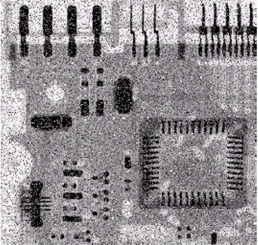2、问题分析

若想改善图片的质量，我们需要去除其中的噪声。图片轮廓不清晰，我们将其锐化即可。

下面简单介绍一下处理过程中遇到的问题及解决方法：

问题

解决方法

噪声

中值滤波

各地区颜色不分明，模糊

分段线性变换

边界不清晰，棱角不分明

图像锐化

二、实验过程

1、中值滤波

（1）函数

medianblur(src , dst , size)

（2）参数说明

src：表示原始图像

dst：表示处理后的图像

size：孔径线性尺寸（必须是大于1的奇数）

（3）函数功能

使用中值滤波来平滑图像，图像去噪

2、分段线性变换

（1）函数

SLT(image, x1, x2, y1, y2)

（2）参数说明

Image : 原始图像

（3）函数功能

使图像有更好的目视效果或突出有用的信息,提高图像质量和突出所需信息

3、图像增强

（1）函数

filter2d(img_path)

（2）参数说明

img_path： 输入图片路径

（3）函数功能

是使图像边缘更清晰的一种图像处理方法

三、实验结果

1、灰度图2、中值滤波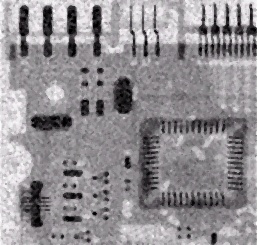3、分段线性变换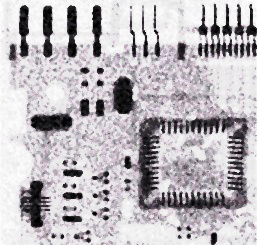4、图像锐化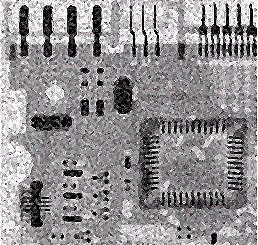5、效果对比图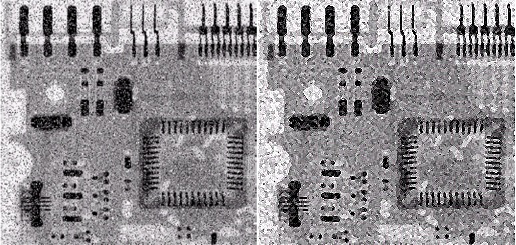四、代码

1、medianBlur.py

import cv2

# 读取图片
img = cv2.imread('img0.png')
pre_img = cv2.cvtColor(img, cv2.COLOR_BGR2RGB)

# 中值滤波
img1 = cv2.medianBlur(img,3)

# 显示并保存图片
cv2.imshow('pre_img',img)
cv2.imshow('medianBlur',img1)
cv2.imwrite('img1.jpg', img1)
cv2.waitKey(0)

2、SLT.py

import numpy as np
import cv2 as cv2

# 读取图片
img = cv2.imread('img1.jpg')
pre_img = cv2.cvtColor(img, cv2.COLOR_BGR2RGB)

def SLT(img, x1, x2, y1, y2):
lut = np.zeros(256)
for i in range(256):
if i < x1:
lut[i] = (y1 / x1) * i
elif i < x2:
lut[i] = ((y2 - y1) / (x2 - x1)) * (i - x1) + y1
else:
lut[i] = ((y2 - 255.0) / (x2 - 255.0)) * (i - 255.0) + 255.0
img2 = cv2.LUT(img, lut)
img2 = np.uint8(img2 + 0.5)
return img2

# 分段线性变换
img2 = SLT(img, 80, 150, 50, 230)

# 显示并保存图片
cv2.imshow('SLT', img2)
cv2.imwrite('img2.jpg', img2)
cv2.waitKey(0)

3、filter2d.py

import cv2 as cv2
import numpy as np

# 读取图像
img = cv2.imread('img1.jpg')
pre_img = cv2.cvtColor(img, cv2.COLOR_BGR2RGB)
img0 = cv2.imread('img0.png')
img0 = cv2.cvtColor(img0, cv2.COLOR_BGR2RGB)

def filter2d(img_path):
kernel = np.array([[0, -1, 0],
[-1, 5, -1],
[0, -1, 0]])

img3 = cv2.filter2D(img, -1, kernel)
return img3

# 图像锐化
img3 = filter2d(img)

merge = np.hstack((img0,img3))

# 显示并保存图像
cv2.imshow('filter2D', img3)
cv2.imwrite('img3.jpg',img3)
cv2.imshow('merge',merge)
cv2.imwrite('merge.jpg',merge)
cv2.waitKey(0)


展开全文opencv 计算机视觉
• 数字图像处理 实验代码 python+opencv exp1图像的显示与存储，exp2基本几何变换，平移、旋转、缩放等,图像的傅里叶变换，exp3图像的对比度增强+直方图修正+平滑、锐化，exp4边缘检测
• 文章目录第二章 数字图像基础1、用程序实现同时对比度实验2、用程序实验空间分辨率变化效果3、用程序实验幅度分辨率变化效果 第二章 数字图像基础 这几个题目网上有很多MATLAB版的解答，这里自己写了一个python的...
• 实验流程转换图 理想带通滤波器代码 #导入工具包 import cv2 import numpy as np import math from matplotlib import pyplot as plt # 读取图像 img = cv2.imread('C:/Users/lenovo/Desktop/lena.jpg', 0)#0...
带通滤波器

概念
允许带内频率对应像素的灰度值通过而阻止带外的

公式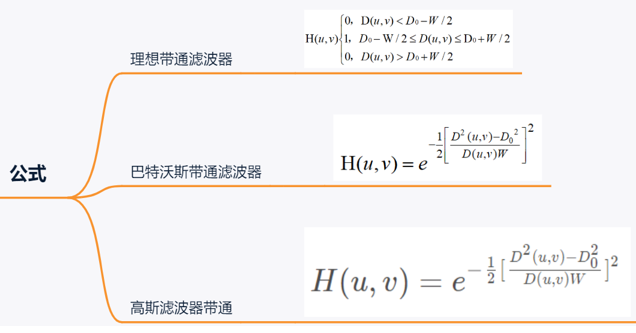公式中的符号含义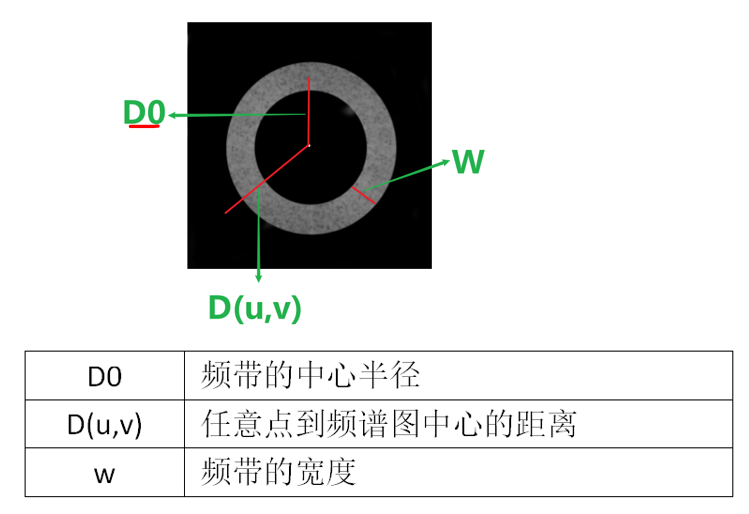实验流程转换图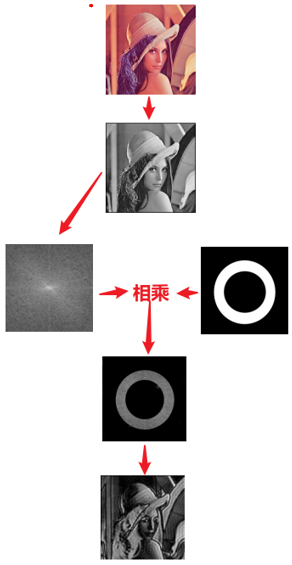理想带通滤波器代码

#导入工具包
import cv2
import numpy as np
import math
from matplotlib import pyplot as plt

# 读取图像
img = cv2.imread('C:/Users/lenovo/Desktop/lena.jpg', 0)#0的含义:将图像转化为单通道灰度图像

def ideal_bandpass_filter(img,D0,w):
img_float32 = np.float32(img)#转换为np.float32格式，这是oppencv官方要求，咱们必须这么做
dft = cv2.dft(img_float32, flags=cv2.DFT_COMPLEX_OUTPUT)#傅里叶变换,得到频谱图
dft_shift = np.fft.fftshift(dft)#将频谱图低频部分转到中间位置，三维(263,263,2)
rows, cols = img.shape #得到每一维度的数量
crow, ccol = int(rows / 2), int(cols / 2)  # 中心位置
mask = np.ones((rows, cols,2), np.uint8)  #对滤波器初始化，长宽和上面图像的一样
for i in range(0, rows): #遍历图像上所有的点
for j in range(0, cols):
d = math.sqrt(pow(i - crow, 2) + pow(j - ccol, 2)) # 计算(i, j)到中心点的距离
if D0 - w / 2 < d < D0 + w / 2:
mask[i, j,0]=mask[i,j,1] = 1
else:
mask[i, j,0]=mask[i,j,1] = 0
f = dft_shift * mask  # 滤波器和频谱图像结合到一起，是1的就保留下来，是0的就全部过滤掉
ishift = np.fft.ifftshift(f) #上面处理完后，低频部分在中间，所以傅里叶逆变换之前还需要将频谱图低频部分移到左上角
iimg = cv2.idft(ishift) #傅里叶逆变换
res = cv2.magnitude(iimg[:, :, 0], iimg[:, :, 1]) #结果还不能看，因为逆变换后的是实部和虚部的双通道的一个结果，这东西还不是一个图像，为了让它显示出来我们还需要对实部和虚部进行一下处理才可以
return res

new_image1=ideal_bandpass_filter(img,D0=6,w=10)
new_image2=ideal_bandpass_filter(img,D0=15,w=10)
new_image3=ideal_bandpass_filter(img,D0=25,w=10)

# 显示原始图像和带通滤波处理图像
title=['Source Image','D0=6','D0=15','D0=25']
images=[img,new_image1,new_image2,new_image3]
for i in np.arange(4):
plt.subplot(2,2,i+1),plt.imshow(images[i],'gray')
plt.title(title[i])
plt.xticks([]),plt.yticks([])
plt.show()



巴特沃斯带通滤波器代码

#导入工具包
import cv2
import numpy as np
from matplotlib import pyplot as plt

#滤波器
def butterworth_bandstop_kernel(img,D0,W,n=1):
assert img.ndim == 2
r,c = img.shape,img.shape
u = np.arange(r)
v = np.arange(c)
u, v = np.meshgrid(u, v) #生成网络点坐标矩阵
low_pass = np.sqrt( (u-r/2)**2 + (v-c/2)**2 ) #相当于公式里的D(u,v),距频谱图矩阵中中心的距离
kernel = 1-(1/(1+((low_pass*W)/(low_pass**2-D0**2))**(2*n))) #变换公式
return kernel

def butterworth_bandpass_filter(img,D0,W,n):
assert img.ndim == 2
kernel = butterworth_bandstop_kernel(img,D0,W,n)  #得到滤波器
gray = np.float64(img)  #将灰度图片转换为opencv官方规定的格式
gray_fft = np.fft.fft2(gray) #傅里叶变换
gray_fftshift = np.fft.fftshift(gray_fft) #将频谱图低频部分转到中间位置
#dst = np.zeros_like(gray_fftshift)
dst_filtered = kernel * gray_fftshift #频谱图和滤波器相乘得到新的频谱图
dst_ifftshift = np.fft.ifftshift(dst_filtered) #将频谱图的中心移到左上方
dst_ifft = np.fft.ifft2(dst_ifftshift) #傅里叶逆变换
dst = np.abs(np.real(dst_ifft))
dst = np.clip(dst,0,255)
return np.uint8(dst)

#读取图像
img = cv2.imread('C:/Users/lenovo/Desktop/lena.jpg', 0)

#得到处理后的图像
new_image1=butterworth_bandpass_filter(img,D0=6,W=10,n=1)
new_image2=butterworth_bandpass_filter(img,D0=15,W=10,n=1)
new_image3=butterworth_bandpass_filter(img,D0=25,W=10,n=1)

# 显示原始图像和带通滤波处理后的图像
title=['Source Image','D0=6','D0=15','D0=25']
images=[img,new_image1,new_image2,new_image3]
for i in np.arange(4):
plt.subplot(2,2,i+1),plt.imshow(images[i],'gray')
plt.title(title[i])
plt.xticks([]),plt.yticks([])
plt.show()


高斯带通滤波器代码

#导入工具包
import cv2
import numpy as np
from matplotlib import pyplot as plt

def gaussian_bandstop_kernel(img,D0,W):
assert img.ndim == 2
r,c = img.shape,img.shape
u = np.arange(r)
v = np.arange(c)
u, v = np.meshgrid(u, v)
low_pass = np.sqrt( (u-r/2)**2 + (v-c/2)**2 )
kernel = 1.0 - np.exp(-0.5 * (((low_pass ** 2 - D0**2) / (low_pass *W + 1.0e-5))**2))
return kernel

def gaussian_bandpass_filter(img,D0=5,W=10):
assert img.ndim == 2
kernel = 1.0 - gaussian_bandstop_kernel(img,D0,W)
gray = np.float64(img)
gray_fft = np.fft.fft2(gray)
gray_fftshift = np.fft.fftshift(gray_fft)
dst = np.zeros_like(gray_fftshift)
dst_filtered = kernel * gray_fftshift
dst_ifftshift = np.fft.ifftshift(dst_filtered)
dst_ifft = np.fft.ifft2(dst_ifftshift)
dst = np.abs(np.real(dst_ifft))
dst = np.clip(dst,0,255)
return np.uint8(dst)

#读取图像
img = cv2.imread('C:/Users/lenovo/Desktop/4.png', 0)

new_image1=gaussian_bandpass_filter(img,D0=6,W=10)
new_image2=gaussian_bandpass_filter(img,D0=15,W=10)
new_image3=gaussian_bandpass_filter(img,D0=25,W=10)

# 显示原始图像和带通滤波处理图像
title=['Source Image','D0=6','D0=15','D0=25']
images=[img,new_image1,new_image2,new_image3]
for i in np.arange(4):
plt.subplot(2,2,i+1),plt.imshow(images[i],'gray')
plt.title(title[i])
plt.xticks([]),plt.yticks([])
plt.show()


实验结果
处理正常图像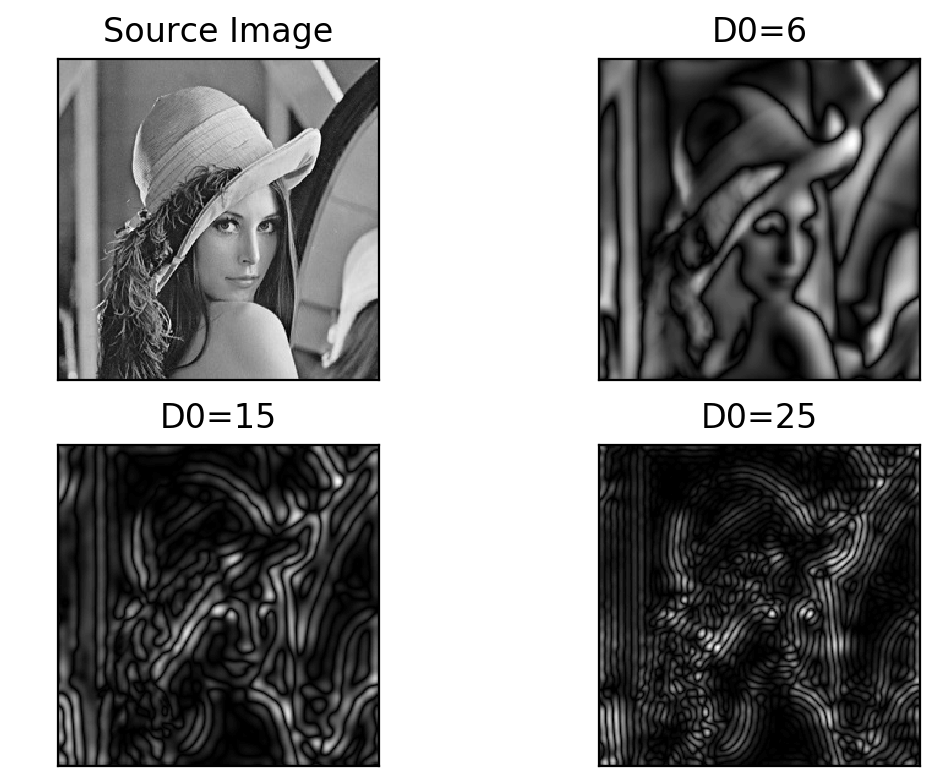处理噪声图像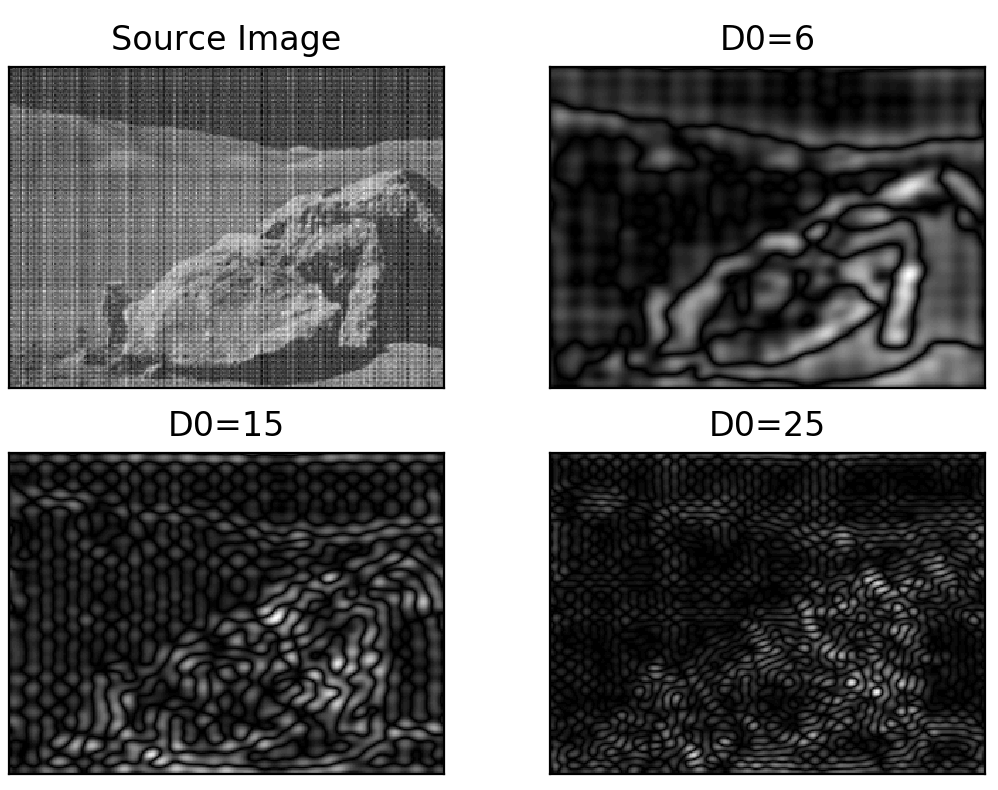实验结论
1、不可以直接使用带通滤波器，如果直接使用会损失大量图像细节
2、可使用带通滤波器提取噪声模式


展开全文计算机视觉 机器学习 数据挖掘 神经网络
• 我们以skimage中的transform模块为例，讲述图像的形变和缩放 实验一：改变图片尺寸: resize from skimage import transform,data import matplotlib.pyplot as plt img = data.astronaut() print ('origin picture ...
• 文章目录第二章 数字图像基础1、用程序实现同时对比度实验2、用程序实验空间分辨率变化效果3、用程序实验幅度分辨率变化效果 第二章 数字图像基础 这几个题目网上有很多MATLAB版的解答，这里自己写了一个python的...
• 图片读取到计算机中，是以numpy数组存在的。 numpy数组的所有功能对图片也适用。 对数组元素的访问就是对图片像素点的访问。 彩色图片访问方式： ...color_img[i,j,c] ...c:图片的通道数（第几通道的意思...实验一：输...
• img2_copy = np.array(pow(img2/255, fGamma) * 255, dtype='uint8')
• 实验一：图像数据类型和转换 在skimage库中，一张图片实际上就是一个简单的numpy数组，数组的数据类型有很多种，相互之间也可以转换。数据类型列举如下： uint8:0-28 uint16:0-216 uint32:0-232 float:[-1,1] or [0,...
• 文章目录第三章 灰度变换与空间滤波1....本章主要介绍了一些在空间域对图像进行处理的技术，空间域就是简单的包含图像像素的平面，空间域技术直接在图像像素上操作。 空间域的处理可由下式进行表示 g(x,y)=T[f(x,y...
• 对于下面这幅图像，编程实现染色体计数，并附简要处理流程说明。 二、实验步骤 1.中值滤波 2.图像二值化 3.膨胀图像 4.腐蚀图像 5.计算光影背景 6.移除背景 7.检测染色体 三、代码 import cv2 import ...
• skimage库提供了io模块，这个模块是用来图片输入输出...实验一：从外部读取图片并显示 #!/usr/bin/env python3 # -*- coding: utf-8 -*- """ Created on Fri May 17 09:51:06 2019 @author: zqq """ # 读取单...
• 文章目录第五章 图像复原与重建1、实现自适应均值滤波，并和算术均值滤波的结果做对比2、实现自适应中值滤波，并和中值滤波的结果做对比3、逆滤波与维纳滤波4、维纳滤波和有约束最小二乘方滤波...第三个和第四个实验...
• 文章目录第四章 频率域滤波1、实现图像的傅里叶变换，显示其幅度谱的图像（0 频在显示图像的中间位置）2、用理想低通滤波器在频率域实现低通滤波3、用理想高通滤波器在频率域实现低通滤波4、用巴特沃斯低通滤波器和...
• 文章目录第八章 图像压缩1、 分别用区域编码和阈值编码方法实现图像压缩 第八章 图像压缩 生命短暂而信息无…缩写是无法避免的灾难，而缩写创造者的任务就是要尽力做好工作。尽管缩写有害,但总比什么也不做要强 1...
• 数字图像处理实验五－图像分割、表示与特征提取 做到旋转的边界矩形时，cv2.cv.BoxPoints()函数处出现'module' object has no attribute 'cv' 的错误 import cv2 import numpy as np img = cv2.imread('leaf.jpg',0......

# 数字图像处理实验pythonpython 订阅